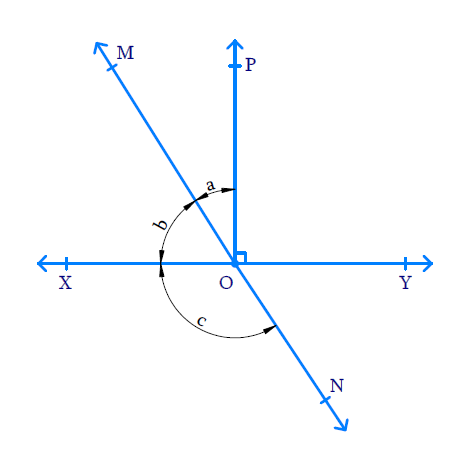# Ex.6.1 Q2 Lines and Angles Solution - NCERT Maths Class 9

Go back to  'Ex.6.1'

## Question

In the given figure, lines $$XY$$ and $$MN$$ intersect at $$O.$$ If $$\angle POY = 90^ {\circ}$$ and $$a:b = 2:3,$$ find $$c.$$Video Solution
Lines And Angles
Ex 6.1 | Question 2

## Text Solution

What is known?

$$\angle POY= 90^ {\circ}$$ and $$a:b = 2:3$$

What is unknown?

$$\angle XON = c =?$$

Reasoning:

If two lines intersect each other, then the vertically opposite angles formed are equal.

Steps:

Line $$OP$$ is perpendicular to line $$XY.$$ Hence $$\angle POY = \angle POX = 90^ {\circ}$$

\begin{align}\angle POX & = \angle POM + \angle MOX\end{align}

\begin{align} 90 ^ { \circ } & = a + b \quad \ldots \ldots . ( 1 ) \end{align}

Since $$a$$ and $$b$$ are in the ratio $$2:3$$ that is, $$a = 2x$$ and $$b = 3x \quad \ldots \ldots . (2)$$

Substituting ($$2$$) in ($$1$$),

\begin{align} a + b &= 90 ^ { \circ } \\ 2 x + 3 x &= 90 ^ { \circ } \\ 5 x &= 90 ^ { \circ } \\ x = \frac { 90 } { 5 } &= 18 ^ { \circ } \\ a = 2 x &= 2 \times 18 ^ { \circ } \\ a &= 36 ^ { \circ } \\ b = 3 x &= 3 \times 18 ^ { \circ } \\ b &= 54 ^ { \circ } \end{align}

Also,\begin{align}\angle MOY & = \angle MOP+ \angle POY\end{align}

\begin{align} & = a + 90 ^ { \circ } \\ & = 36 ^ { \circ } + 90 ^ { \circ } \\&= 126 ^ { \circ } \end{align}

Lines $$MN$$ and $$XY$$ intersect at point $$O$$ and the vertically opposite angles formed are equal.

\begin{align}\angle XON &= \angle MOY \\ c &=126^ {\circ} \end{align}

Video Solution
Lines And Angles
Ex 6.1 | Question 2

Learn from the best math teachers and top your exams

• Live one on one classroom and doubt clearing
• Practice worksheets in and after class for conceptual clarity
• Personalized curriculum to keep up with school